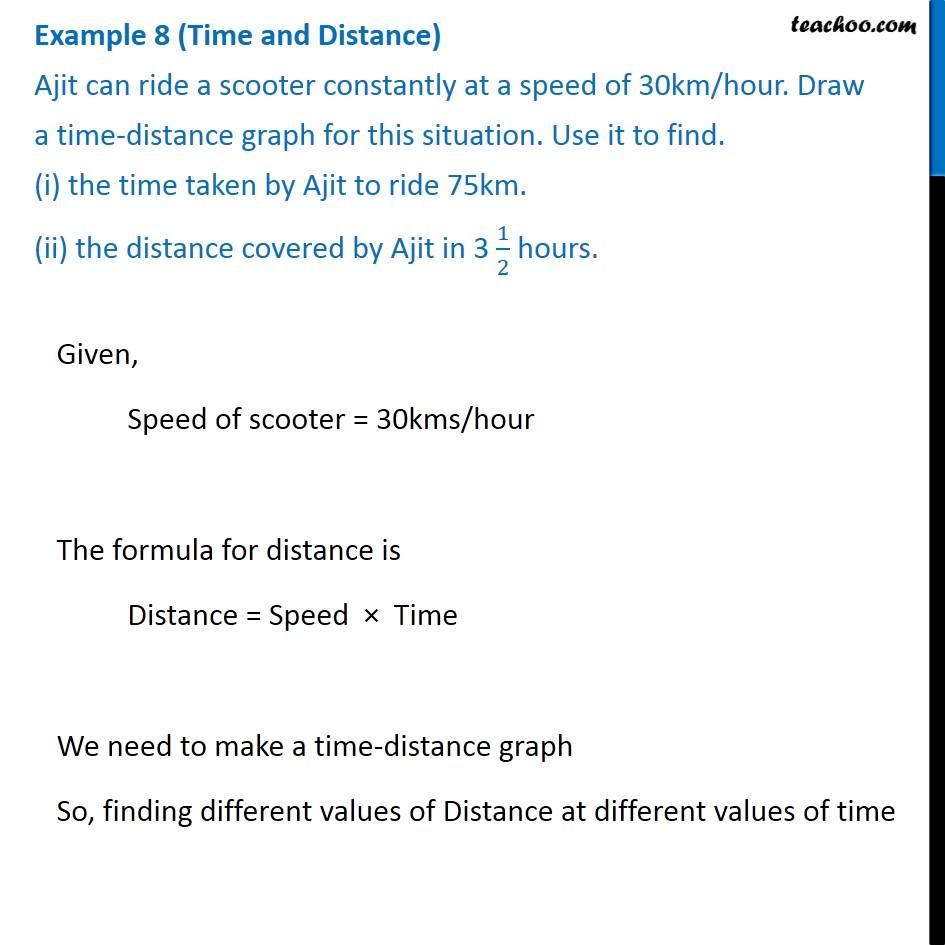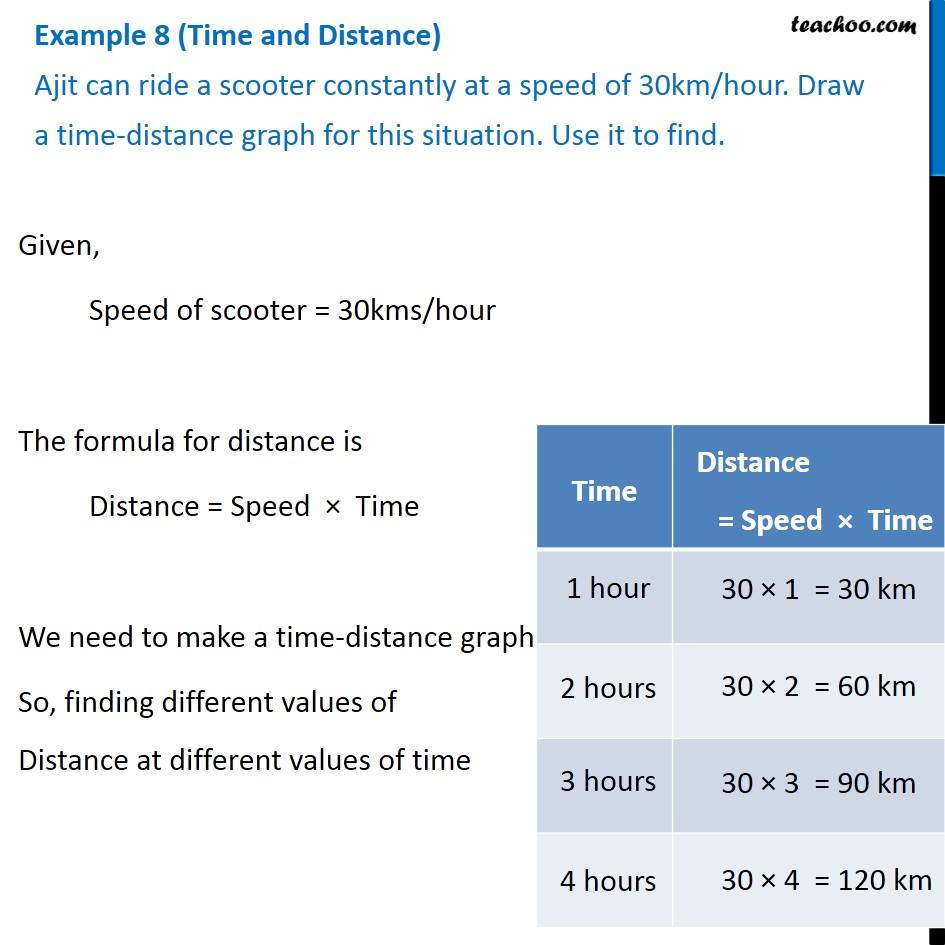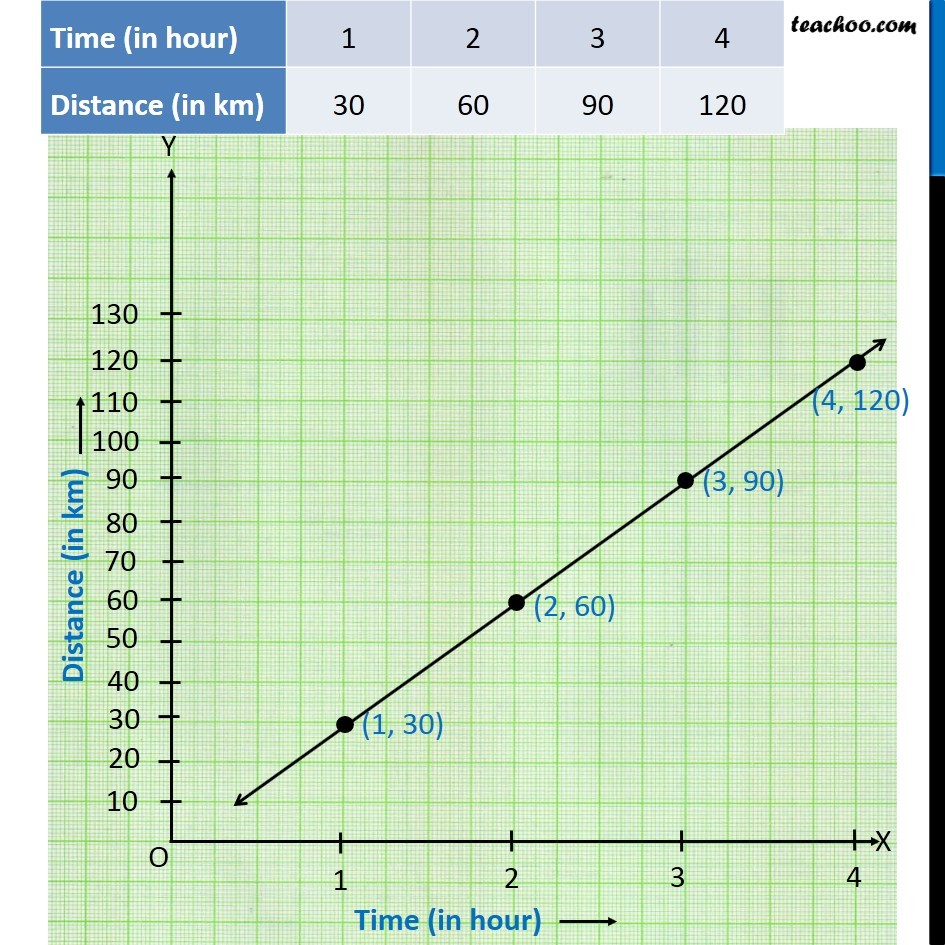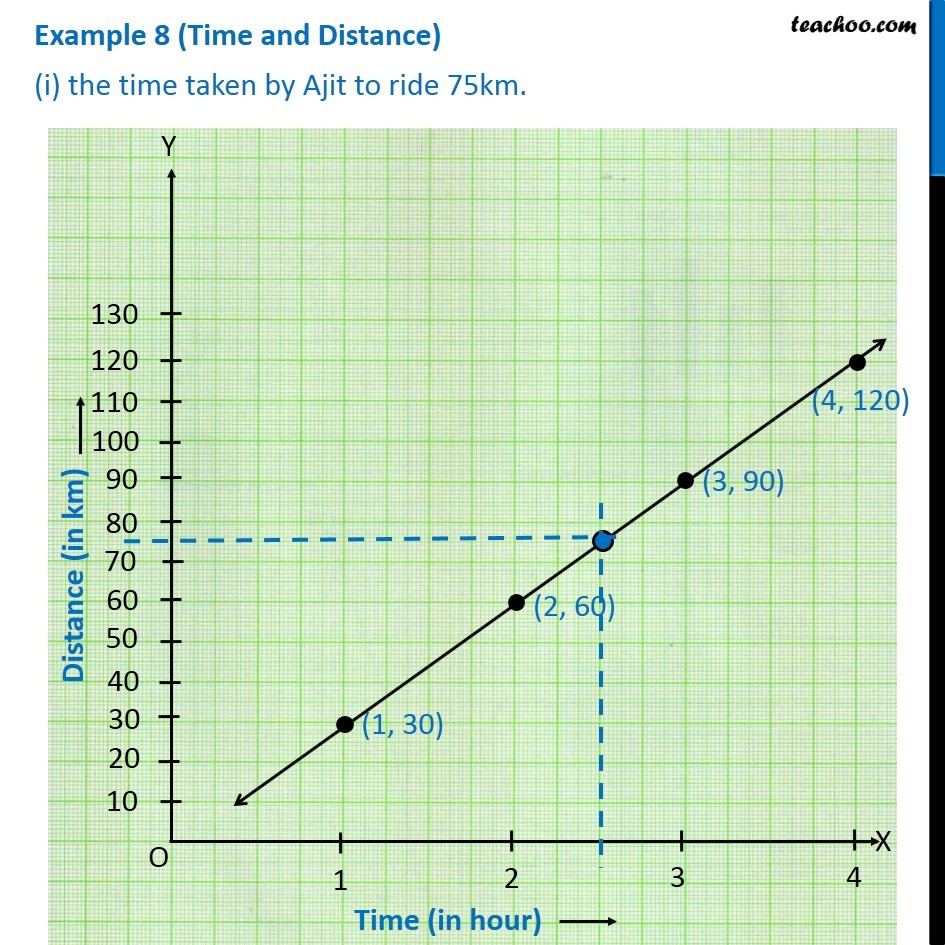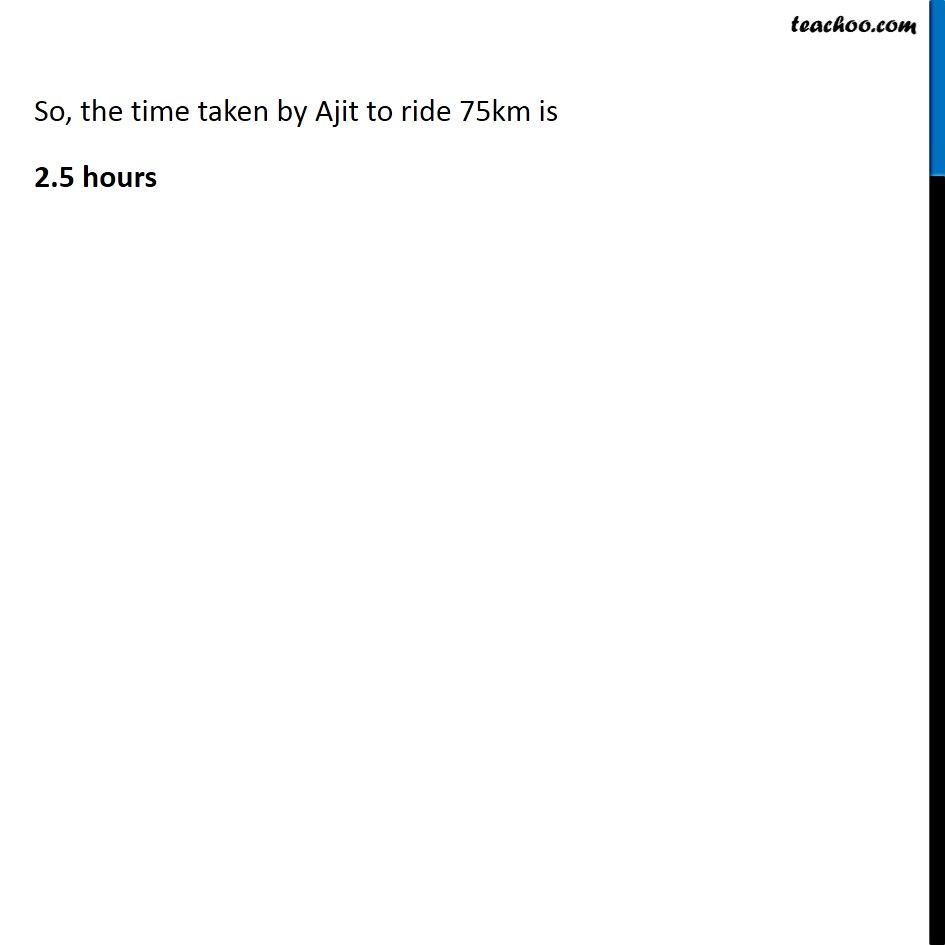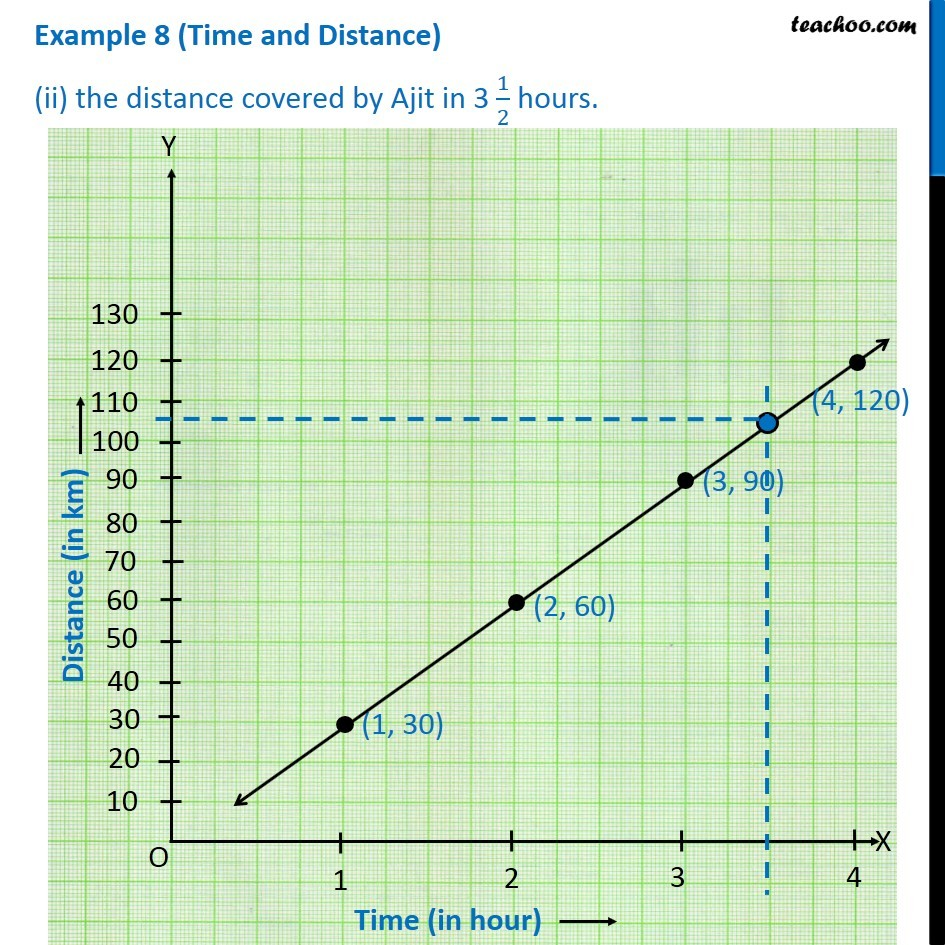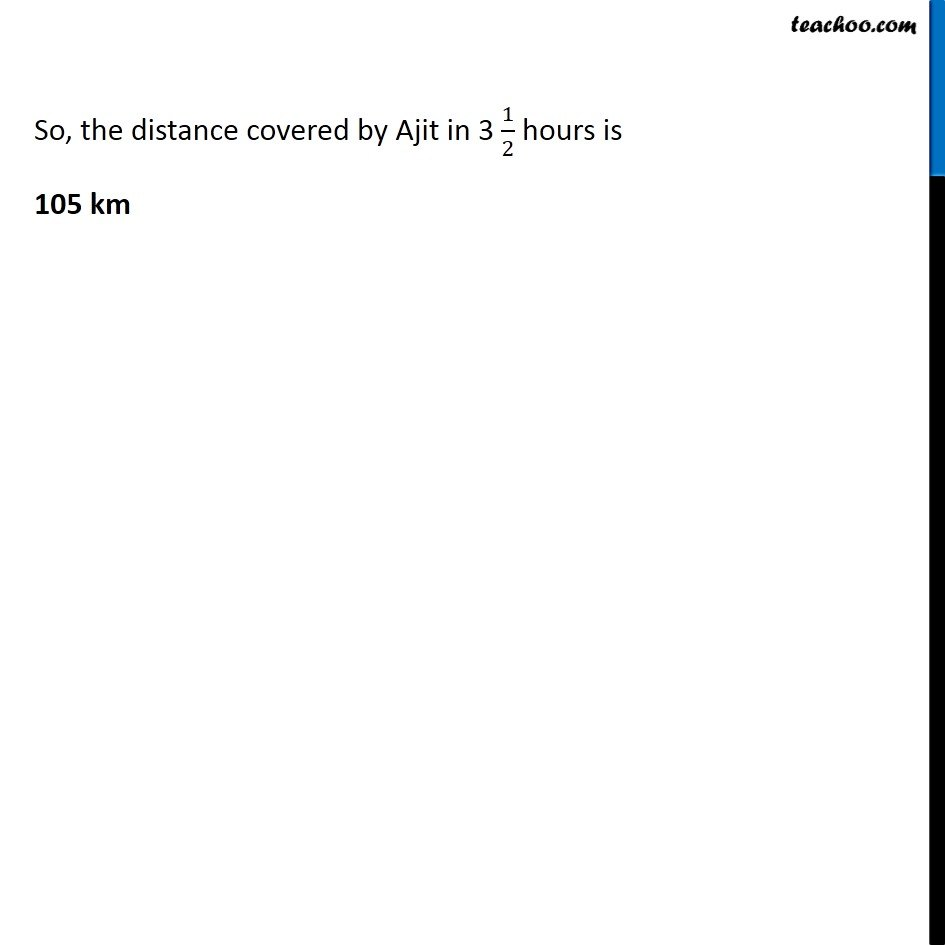Subscribe to our Youtube Channel - https://you.tube/teachoo

1. Chapter 15 Class 8 Introduction to Graphs
2. Concept wise
3. Making Linear Graphs

Transcript

Example 8 (Time and Distance) Ajit can ride a scooter constantly at a speed of 30km/hour. Draw a time-distance graph for this situation. Use it to find. (i) the time taken by Ajit to ride 75km. (ii) the distance covered by Ajit in 3 1/2 hours. Given, Speed of scooter = 30kms/hour The formula for distance is Distance = Speed × Time We need to make a time-distance graph So, finding different values of Distance at different values of time Example 8 (Time and Distance) Ajit can ride a scooter constantly at a speed of 30km/hour. Draw a time-distance graph for this situation. Use it to find. Given, Speed of scooter = 30kms/hour The formula for distance is Distance = Speed × Time We need to make a time-distance graph So, finding different values of Distance at different values of time Example 8 (Time and Distance) (i) the time taken by Ajit to ride 75km. So, the time taken by Ajit to ride 75km is 2.5 hours Example 8 (Time and Distance) (ii) the distance covered by Ajit in 3 1/2 hours. So, the distance covered by Ajit in 3 1/2 hours is 105 km

Making Linear Graphs